Function Repository Resource:

# NumberExpansion

Express a number as an expansion using any of several methods

Contributed by: Swastik Banerjee
 ResourceFunction["NumberExpansion"][x,n,t] generates a list of the first n terms in the series representation of x for the chosen expansion type t. ResourceFunction["NumberExpansion"][x,t] generates a list of all the terms that can be obtained using arbitrary-precision arithmetic.

## Details and Options

"Lüroth" can also be written as "Lueroth" when passed as an argument.
The Engel expansion representation {a1,a2,a3,} corresponds to the expression 1/a1+1/a1a2+1/a1a2a3+.
The Pierce expansion (alternating Engel expansion) representation {a1,a2,a3,} corresponds to the expression 1/a1-1/a1a2+1/a1a2a3-.
The Sylvester expansion representation {a1,a2,a3,} corresponds to the expression 1/a1+1/a2+1/a3+.
The Cantor expansion representation {a1,a2,a3,} corresponds to the expression a1*1!+a2*2!+a3*3!+.
The Cantor product expansion representation {a1,a2,a3,} corresponds to the expression (1+1/a1)(1+1/a2)(1+1/a3).
The Lüroth expansion representation {a1,a2,a3,} corresponds to the expression 1/a1 + 1/a1(a1-1)a2 + 1/a1(a1-1)a2(a2-1)a3+.
The Oppenheim expansion representation {d1,d2,d3,} corresponds to the expression 1/d1+(a1/b1)(1/d2)+(a1a2/b1b2)(1/d2)+f.
The Oppenheim expansion requires explicit specification of the constants a and b as defined in the original paper by A. Oppenheim. ResourceFunction["NumberExpansion"][x, n, r, s, p, q, "Oppenheim"] generates a list of the first n terms in the Oppenheim series representation of x where ai=r+s*di and bi=p+q*di , i=1,2,.,n.
The x can be either an exact or an inexact number.
For exact numbers, ResourceFunction["NumberExpansion"][x, t ] can be used if x is rational.
Since irrational numbers always yield an infinite sequence, the number of terms has to be specified explicitly.
Since the series expansion representation for a rational number has only a limited number of terms, ResourceFunction["NumberExpansion"][x, n, t] may yield a list with fewer than n elements in this case.
Lüroth expansion always gives a terminating sequence, or an infinite periodic sequence for rational numbers. The latter is represented as {p,{a1,a2,a3,,ap}}, where p is the periodicity.
Resource function FromNumberExpansion reconstructs a number from the result of ResourceFunction["NumberExpansion"].

## Examples

### Basic Examples (1)

10 terms in the Engel expansion of π:

 In:=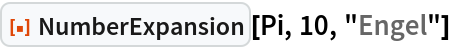Out=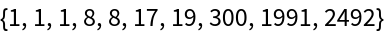### Scope (8)

Expand a rational number:

 In:=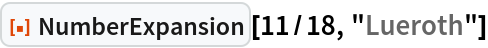Out=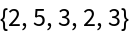Compute the original value using the definition of the expansion:

 In:=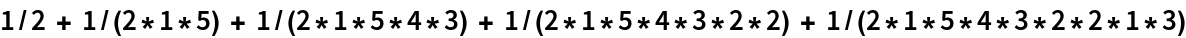Out=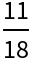Engel expansion of the number 1.175:

 In:=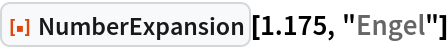Out=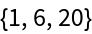First 5 terms of the Pierce expansion of the number 1/√2:

 In:=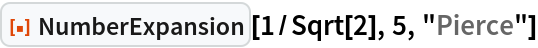Out=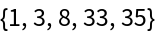Sylvester expansion of the rational number 3/19:

 In:=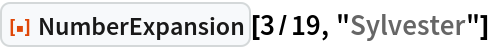Out=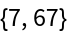Cantor expansion of the number 384:

 In:=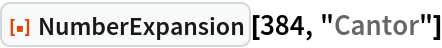Out=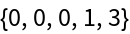First 5 terms of the Cantor product expansion of the irrational number π:

 In:=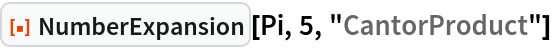Out=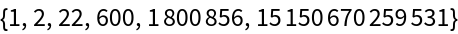Lüroth expansion of the rational number 5/13. It returns an infinite expansion series with periodicity 3:

 In:=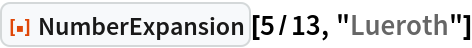Out=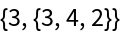Lüroth expansion of the golden ration reciprocal:

 In:=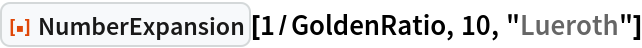Out=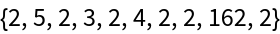Oppenheim expansion of 1/π. See Details and Options for the extra parameters in the Oppenheim expansion:

 In:=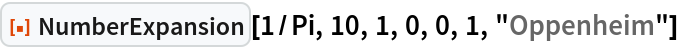Out=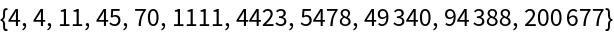### Applications (2)

Fractions of the form a/3k are conjectured to always have a finite Lüroth expansion. This can be investigated with the following:

 In:=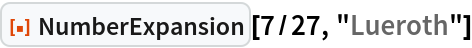Out=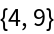In:=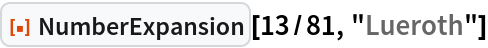Out=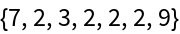Whether all terms of the Sylvester series (Sylvester expansion of the number 1) are square-free is an open problem. All known terms till now are square-free. This urges us to further investigate which terms might have all square-free terms. The Sylvester expansions for 1/Pi and have square terms:

 In:=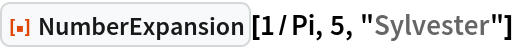Out=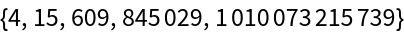In:=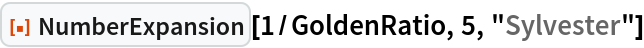Out=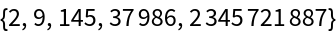From this, it might seem numbers greater than or equal to 1 might have all square-free terms. But the Sylvester expansion of ϕ seems to have a square term:

 In:=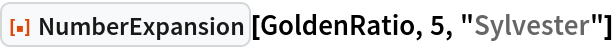Out=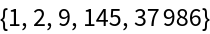### Properties and Relations (1)

The resource function FromNumberExpansion is effectively the inverse of NumberExpansion:

 In:=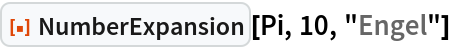Out=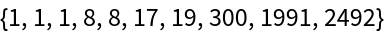In:=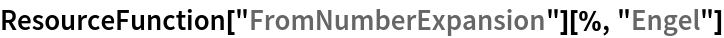Out=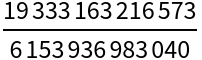In:=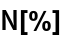Out=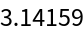### Possible Issues (5)

Expanding an irrational number requires specifying a finite length:

 In:=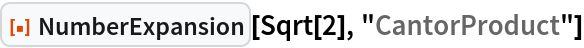Out=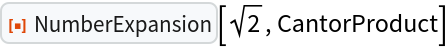In:=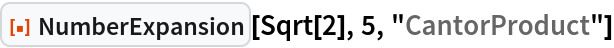Out=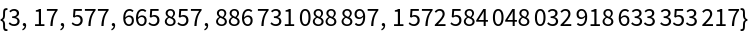In:=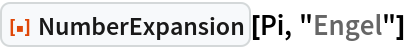Out=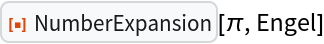In:=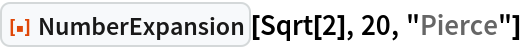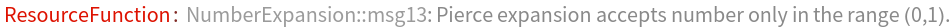Out=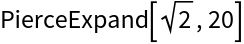In:=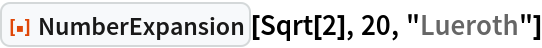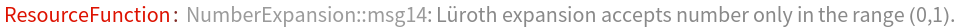Out=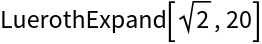Since the function uses arbitrary-precision arithmetic, computing a large number of terms might be very slow depending on the user's hardware, or might run past the limit imposed by \$MaxExtraPrecision:

 In:=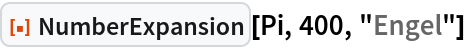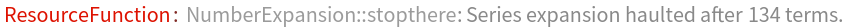Out=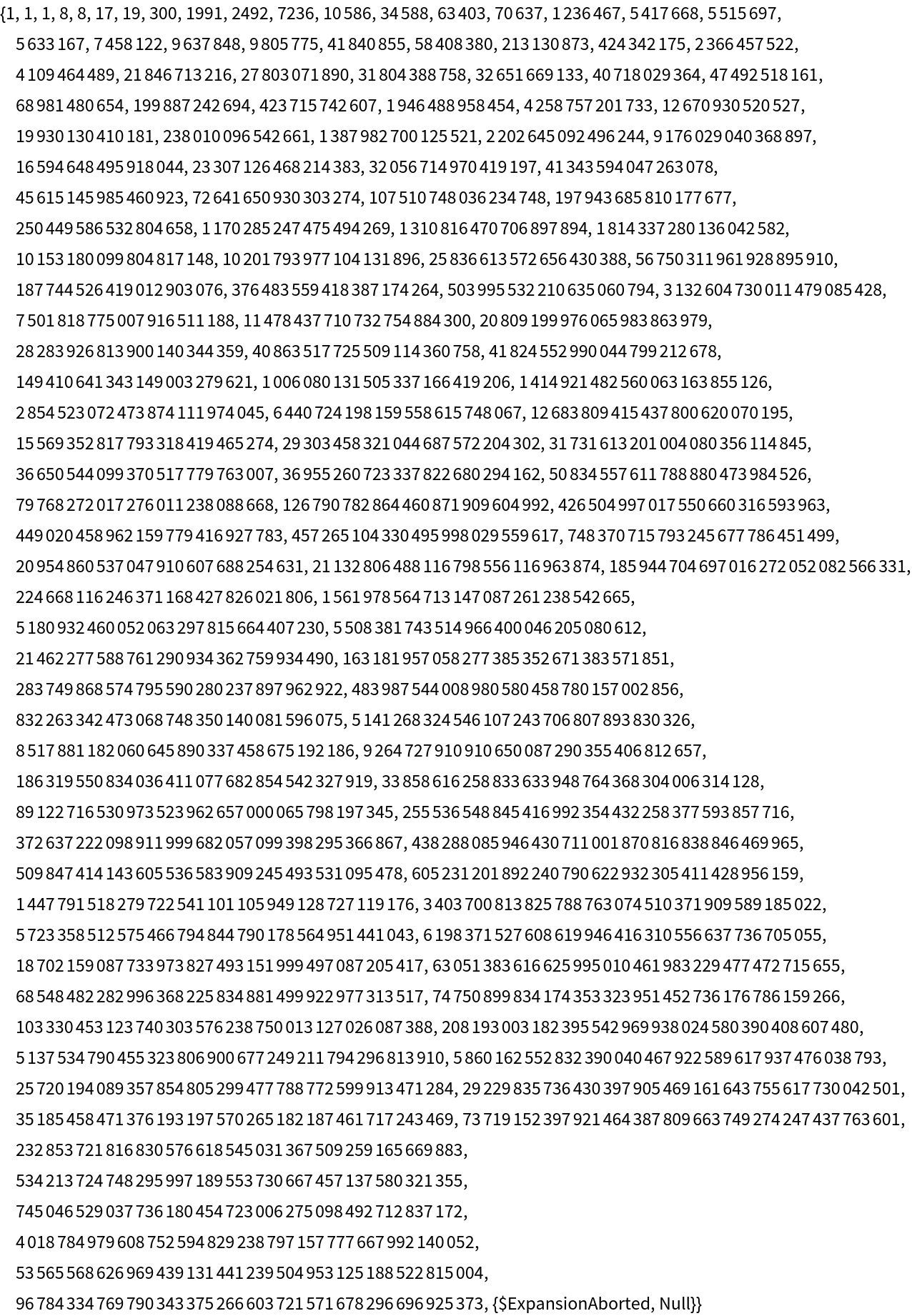## Publisher

Wolfram Summer School

## Version History

• 1.0.0 – 14 September 2020

## Author Notes

Only 2 or 3 test cases seem to give inconsistent results for Cantor product expansion, like 1/Pi, 1/GoldenRatio and 1/Sech. Otherwise, all other test cases seem to give consistent results after rigorous testing on varied test cases.